# Quantity based Quiz for IBPS , SBI , RBI: Quiz – 2

## Quantity based Quiz for IBPS , SBI , RBI: Quiz – 2

Quantity Based Questions are one of the topics of Quantitative Aptitude Section that is asked in government exams. Understanding all the concepts is essential to answer the Quantity Based Questions. In this article, we have come up with the Quantity Based Quiz to enhance your preparation. This Quantity Based Quiz is available to candidates for free. This Quantity Based Quiz includes questions ranging from simple to difficult. A full explanation for each question will be available in this Quantity Based Quiz. This Quantity Based Quiz will help candidates to achieve good scores in their upcoming examinations.

Directions (1-10): In the given questions, two quantities are given, one as Quantity I and another as Quantity II. You have to determine relationship between two quantities and choose the appropriate option.

1. B is thrice as efficient as C. B and C can compete a work together in days. A takes 50% more days than the days taken by A and B to complete the same work together.

Quantity I: No. of days taken by fastest among them to complete the work alone.

Quantity II: Time taken by A and C to complete the work together.

(a) Quantity I > Quantity II

(b) Quantity I < Quantity II

(c) Quantity I ≥ Quantity II

(d) Quantity I ≤ Quantity II

(e) Quantity I = Quantity II or No relation1. Quantity I The profit earned by selling an item (in Rs.) if the difference b/w the SP and the CP is 117 2/3% of Rs.600.

Quantity II The cost price of an article (in Rs.) if the selling price of the article is Rs.1000 and he got 25% profit after selling the item.

(a) Quantity I > Quantity II

(b) Quantity I < Quantity II

(c) Quantity I ≥ Quantity II

(d) Quantity I ≤ Quantity II

(e) Quantity I = Quantity II or No relation1.(a) Quantity I > Quantity II

(b) Quantity I < Quantity II

(c) Quantity I ≥ Quantity II

(d) Quantity I ≤ Quantity II

(e) Quantity I = Quantity II or No relation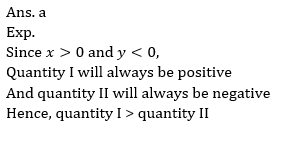2. The largest possible right circular cylinder is cut out from a wooden cube of edge 7 cm.

Quantity I: volume of the cube left over after cutting out the cylinder

Quantity II: Total Surface area of cube remained after cutting out the cylinder.

Note: compare the magnitudes of both quantities.

(a) Quantity I > Quantity II

(b) Quantity I < Quantity II

(c) Quantity I ≥ Quantity II

(d) Quantity I ≤ Quantity II

(e) Quantity I = Quantity II or No relation1. Quantity I: difference between the largest and the smallest part of the sum. A sum of Rs.1440 is lent out in three parts in such a way that the simple interests on first part at 2% for 3 years, second part at 3% for 4 years and third part at 4% for 5 years are equal.

Quantity II: Rs.460

(a) Quantity I > Quantity II

(b) Quantity I < Quantity II

(c) Quantity I ≥ Quantity II

(d) Quantity I ≤ Quantity II

(e) Quantity I = Quantity II or No relation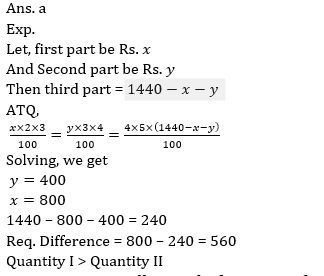1. Quantity I : A seller marked up price of a table 70% above and give two discount of 20% and 25% respectively on M.P. if seller make a profit of 75 Rs. Then find the CP of table?

Quantity II : Cost price of a table is 35% more than cost price of a chair, if shopkeeper sold chair at 25% profit and table at  of loss he gets the total loss of 1350 Rs. find the cost price of table?

(a) Quantity I > Quantity II

(b) Quantity I < Quantity II

(c) Quantity I ≥ Quantity II

(d) Quantity I ≤ Quantity II

(e) Quantity I = Quantity II or No relation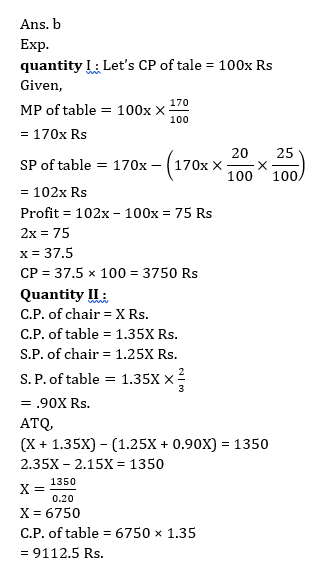1. Quantity I: Satish took education loan of 75000 Rs from SBI at the rate of 10% compound annually, if after 2 year he paid an amount of 30750, then find how much more amount Satish have to pay for completing his loan in next two years?

Quantity II: A and B started a business in partnership with the investment of Rs. 27000 and Rs. 36000 respectively, after 4-month A withdraw 5000 Rs. and B added 6000 Rs. more and C joined with 35000 Rs. if after one year they get a total profit of Rs. 130500, then find the profit share of C ?

(a) Quantity I > Quantity II

(b) Quantity I < Quantity II

(c) Quantity I ≥ Quantity II

(d) Quantity I ≤ Quantity II

(e) Quantity I = Quantity II or No relation1. In a two-digit number, digit at unit place exceeds the digit in its tens place by 2 and the product of the required number with the sum of its digit is equal to 144.

Quantity I: Value of two-digit number

Quantity II: 26

(a) Quantity I > Quantity II

(b) Quantity I < Quantity II

(c) Quantity I ≥ Quantity II

(d) Quantity I ≤ Quantity II

(e) Quantity I = Quantity II or No relation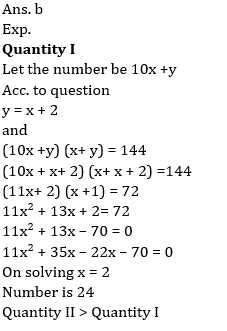1. Quantity I: Percentage profit in real earned by the shopkeeper if at the time of selling and purchasing he used false weights weighing 10% less and 20% more per kilogram respectively and then sold goods at 5% profit.

Quantity II: ‘x’ ; A book was sold for a certain sum and there was a loss of 20%. Had it been sold for Rs 12 more, there would have been a gain of 30%. ‘x’ would be value of profit percent if the book were sold for Rs 4.8 more than what it was sold for in starting.

(a) Quantity I > Quantity II

(b) Quantity I < Quantity II

(c) Quantity I ≥ Quantity II

(d) Quantity I ≤ Quantity II

(e) Quantity I = Quantity II or No relation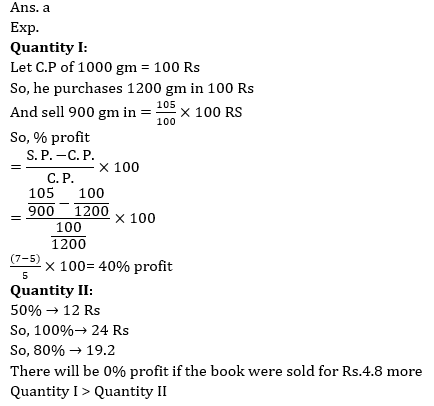1. When a 30% discount is given on marked price of an article, 20% profit is earned. If article is sold for Rs 35 more, then profit percent increases by 50%.

Quantity I: Selling price of article after Rs 35 more is added.

Quantity II: Selling price of article when it sold at 24% discount.

(a) Quantity I > Quantity II

(b) Quantity I < Quantity II

(c) Quantity I ≥ Quantity II

(d) Quantity I ≤ Quantity II

(e) Quantity I = Quantity II or No relation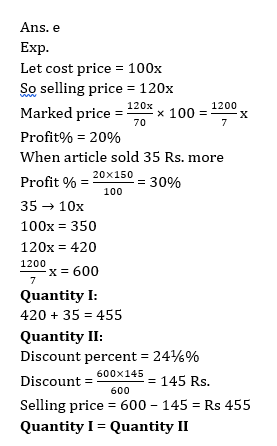#### Attempt Quantitative Aptitude Topic Wise Online Test Series

Recommended PDF’s for:

#### Most important PDF’s for Bank, SSC, Railway and Other Government Exam : Download PDF Now

AATMA-NIRBHAR Series- Static GK/Awareness Practice Ebook PDF Get PDF here
The Banking Awareness 500 MCQs E-book| Bilingual (Hindi + English) Get PDF here
AATMA-NIRBHAR Series- Banking Awareness Practice Ebook PDF Get PDF here
Computer Awareness Capsule 2.O Get PDF here
AATMA-NIRBHAR Series Quantitative Aptitude Topic-Wise PDF Get PDF here
AATMA-NIRBHAR Series Reasoning Topic-Wise PDF Get PDF Here
Memory Based Puzzle E-book | 2016-19 Exams Covered Get PDF here
Caselet Data Interpretation 200 Questions Get PDF here
Puzzle & Seating Arrangement E-Book for BANK PO MAINS (Vol-1) Get PDF here
ARITHMETIC DATA INTERPRETATION 2.O E-book Get PDF here

3